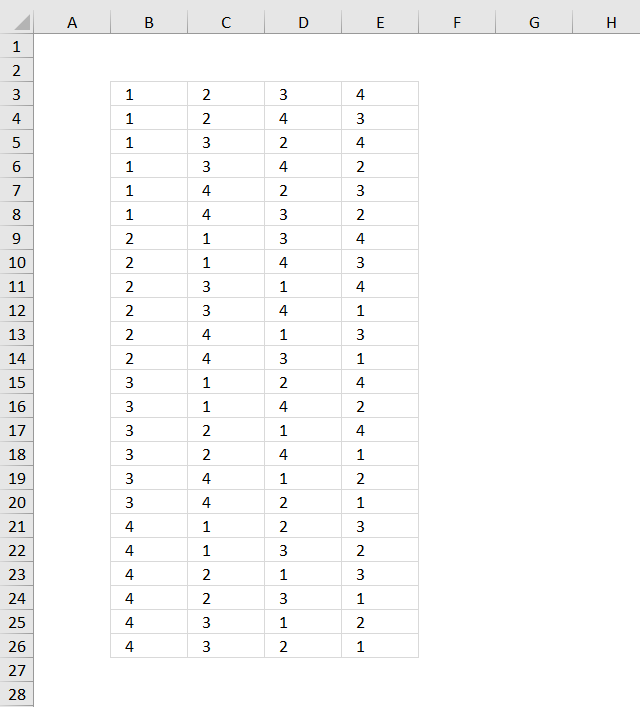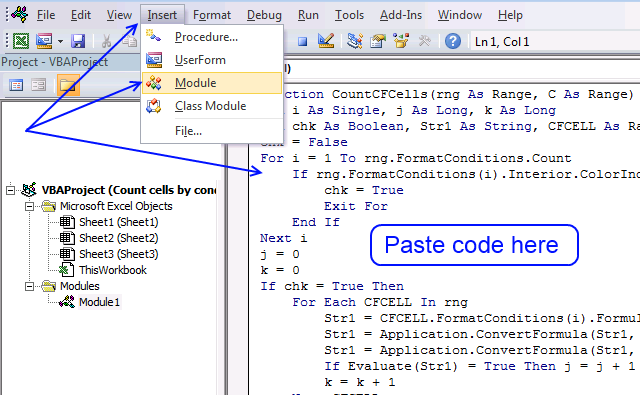Author: Oscar Cronquist Article last updated on January 11, 2019This blog post describes how to create permutations, repetition is NOT allowed. Permutations are items arranged in a given order meaning the order is important.

Examples of permutations are phone numbers, if you enter the digits in the wrong order you might phone someone else, however, phone numbers may have digits repeated and in that case repetition is allowed.

The image above demonstrates how to create permutations of a given number of items and repetition is not allowed, using a user defined function. The numbers are not repeated row-wise.

### How to add the User defined Function to your workbook1. Copy the user defined function below
2. Press Alt-F11 to open the Visual Basic editor
3. Click Module on the Insert menu
4. Paste to code module
5. Exit visual basic editor and go back to Excel
Note: Save your workbook with file extension *.xlsm (Macro-enabled workbook) to keep the function attached to your workbook.

### VBA code

```Function ListPermut(num As Integer)
'Permutations without repetition

Dim c As Long, r As Long, p As Long
Dim rng() As Long, temp As Long, i As Long
Dim temp1 As Long, y() As Long, d As Long
p = WorksheetFunction.Permut(num, num)

' Create array
ReDim rng(1 To p, 1 To num)

'Create first row in array (1, 2, 3, ...)
For c = 1 To num
rng(1, c) = c
Next c

For r = 2 To p
' 1. Find the first smaller number rng(r-1, c-1)<rng(r-1,c)
For c = num To 1 Step -1
If rng(r - 1, c - 1) < rng(r - 1, c) Then
temp = c - 1
Exit For
End If
Next c
' Copy values from previous row
For c = num To 1 Step -1
rng(r, c) = rng(r - 1, c)
Next c
' 2. Find a larger number than rng(r-1, temp)as far to the right as possible
For c = num To 1 Step -1
If rng(r - 1, c) > rng(r - 1, temp) Then
temp1 = rng(r - 1, temp)
rng(r, temp) = rng(r - 1, c)
rng(r, c) = temp1
ReDim y(num - temp)
i = 0
For d = temp + 1 To num
y(i) = rng(r, d)
i = i + 1
Next d
i = 0
For d = num To temp + 1 Step -1
rng(r, d) = y(i)
i = i + 1
Next d
Exit For
End If
Next c
Next r

ListPermut = rng

End Function
```

### How to enter the user defined function

1. Select cell range B3:E26, see top image above.
2. Type =ListPermut(4).
3. Enter the user defined function as an array formula.
1. Press and hold CTRL + SHIFT simultaneously.
2. Now press Enter once.
3. Release all keys.

The formula bar now shows the formula with a beginning and ending curly bracket telling you that you entered the formula successfully. Don't enter the curly brackets yourself.

### Download Excel file through email

Enter your email address to receive the workbook. Note, an email will actually be sent to you.

* You will also get a weekly newsletter, unsubscribe anytime!